Ex 13.2

Chapter 13 Class 10 Surface Areas and Volumes
Serial order wise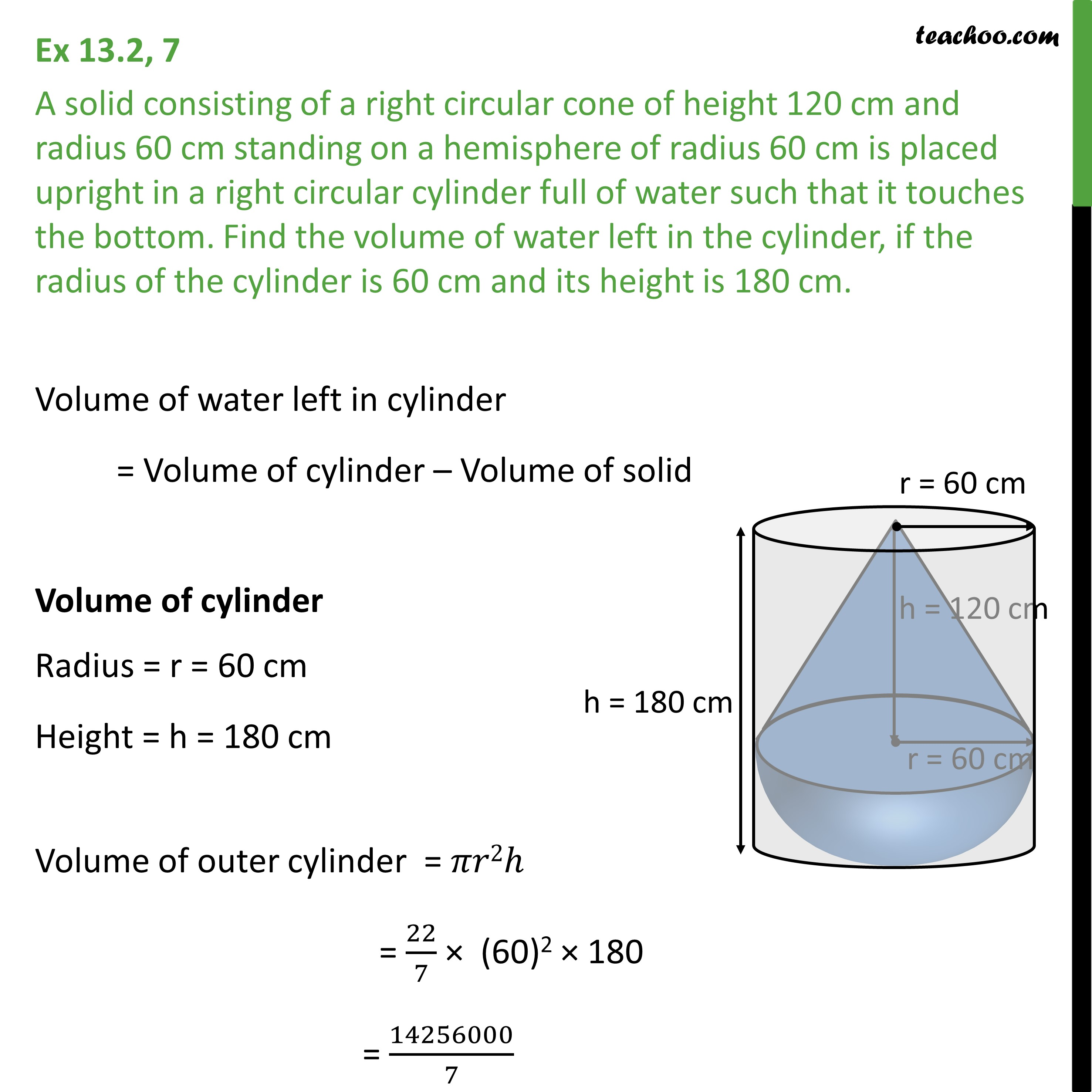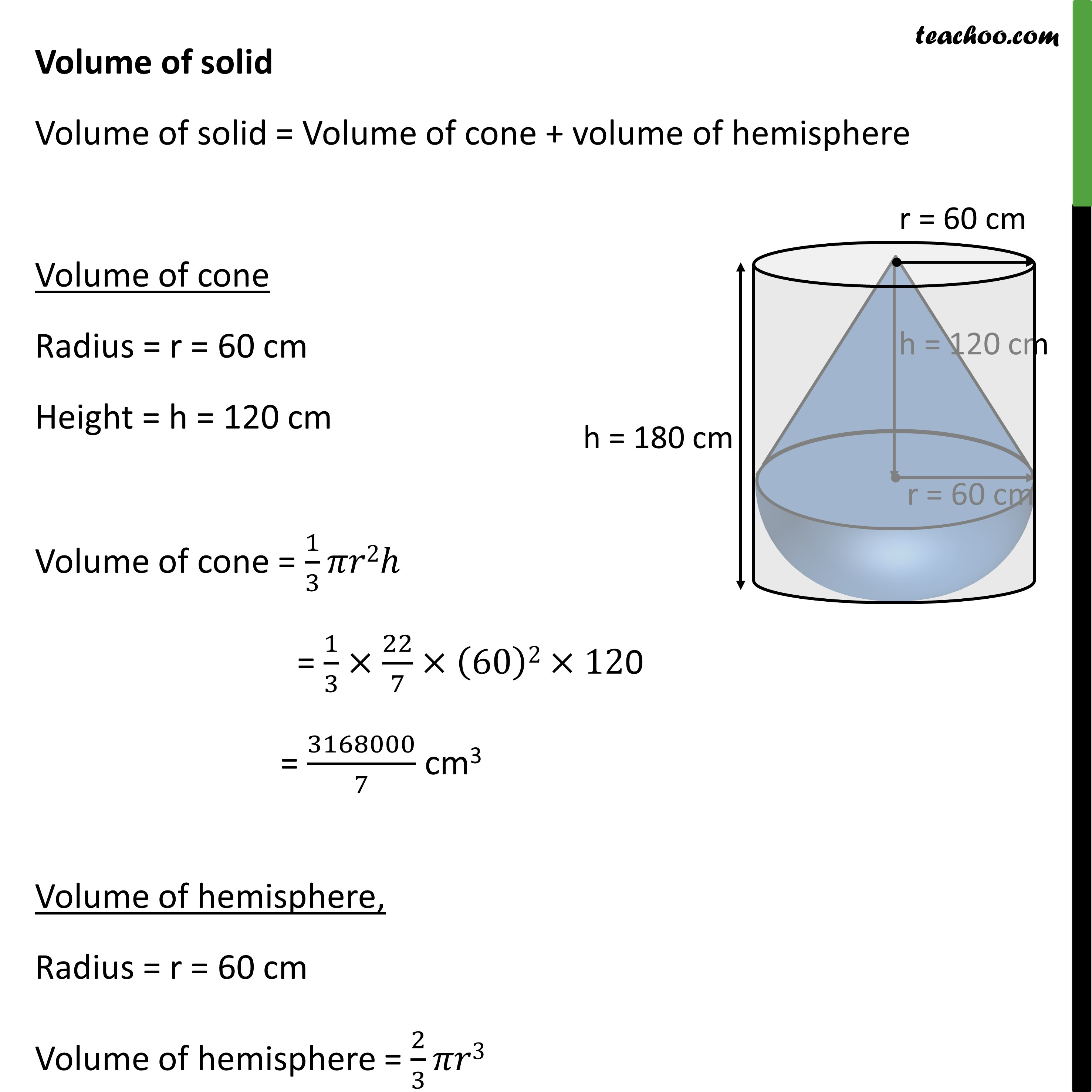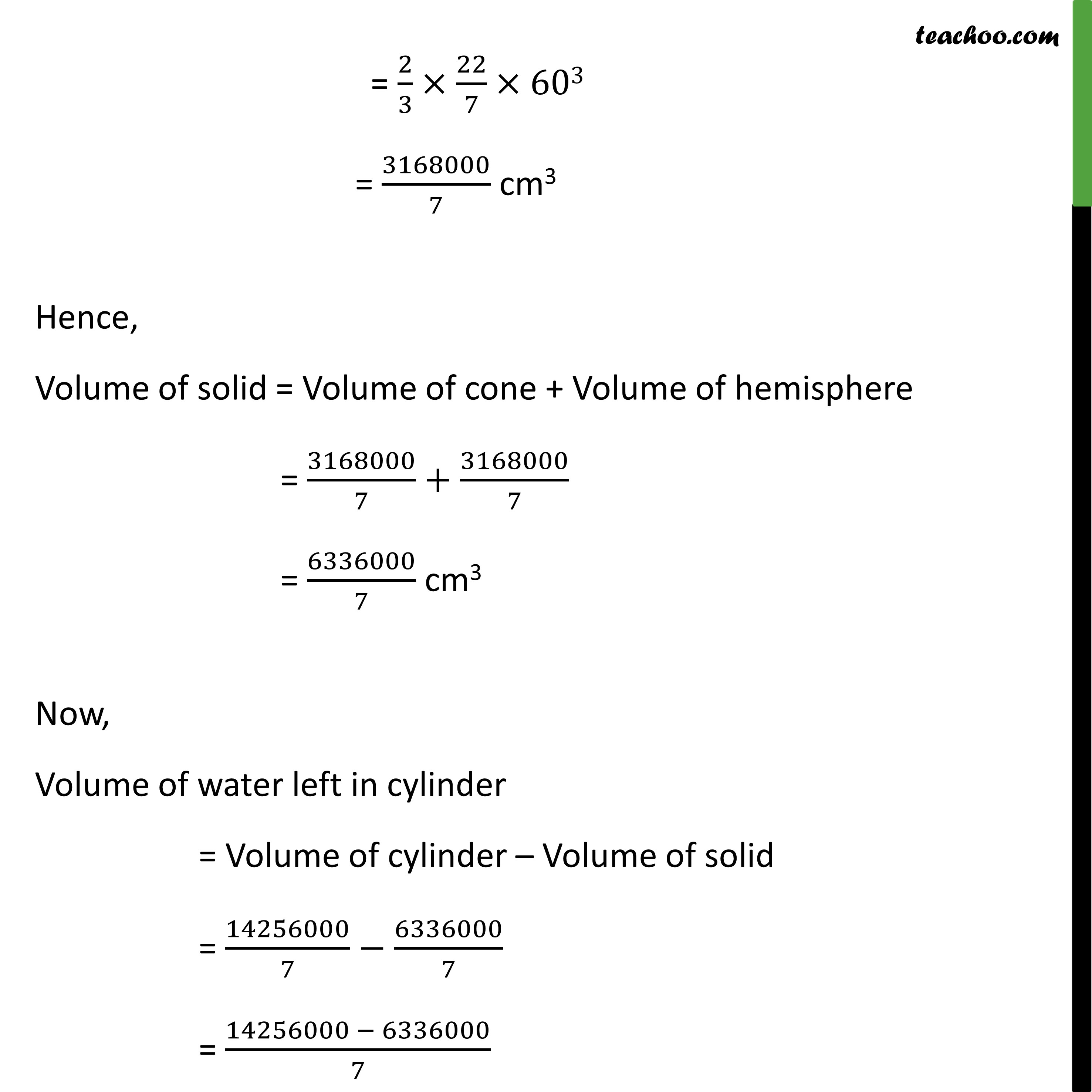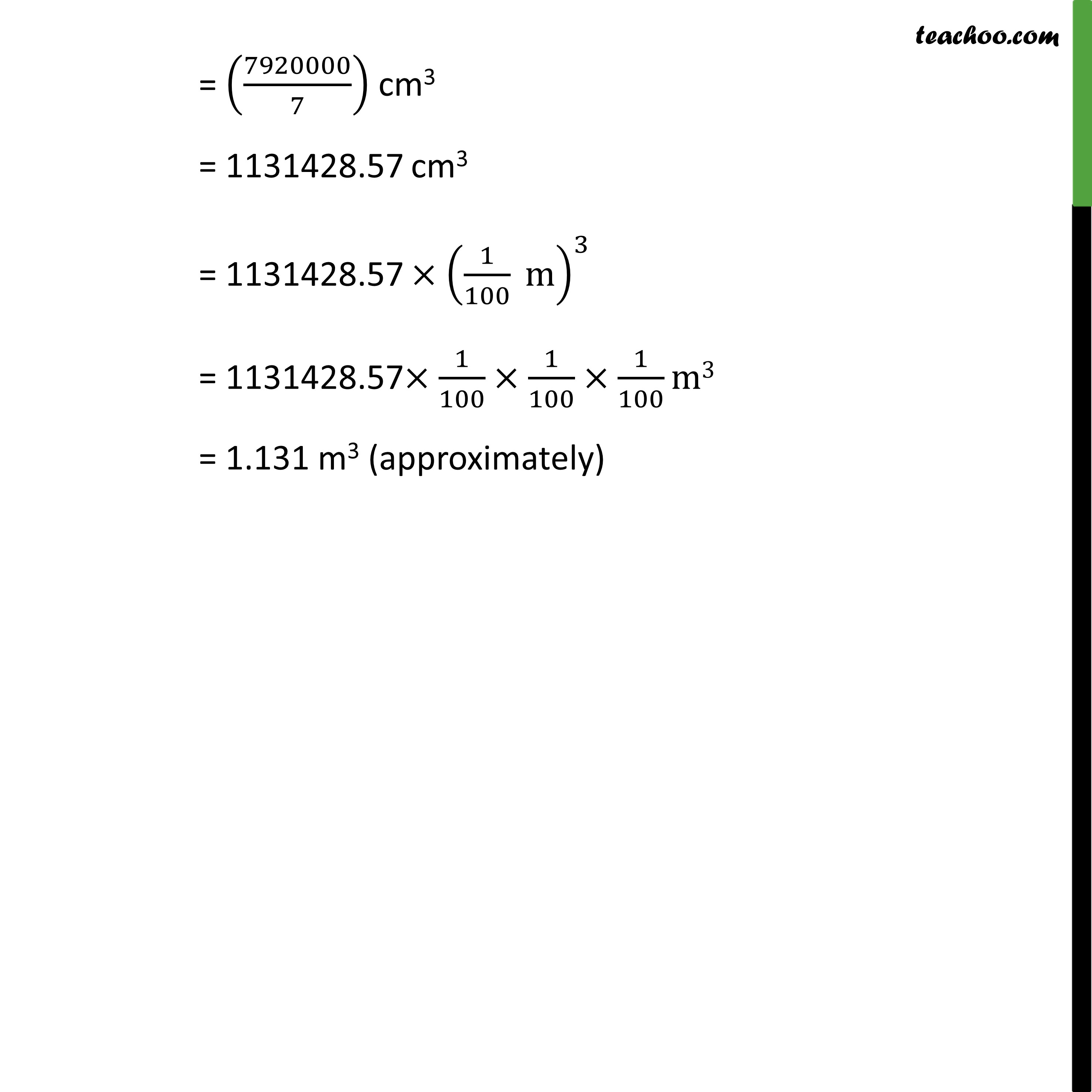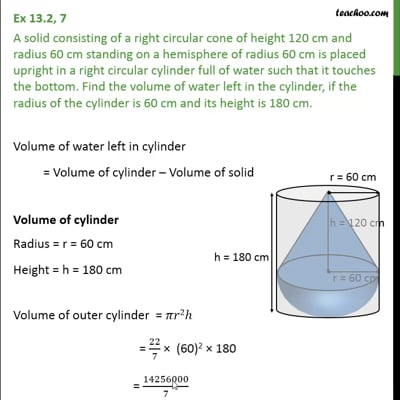This video is only available for Teachoo black users

Maths Crash Course - Live lectures + all videos + Real time Doubt solving!

### Transcript

Ex 13.2, 7 A solid consisting of a right circular cone of height 120 cm and radius 60 cm standing on a hemisphere of radius 60 cm is placed upright in a right circular cylinder full of water such that it touches the bottom. Find the volume of water left in the cylinder, if the radius of the cylinder is 60 cm and its height is 180 cm. Volume of water left in cylinder = Volume of cylinder Volume of solid Volume of cylinder Radius = r = 60 cm Height = h = 180 cm Volume of outer cylinder = 2 = 22/7 (60)2 180 = 14256000/7 Volume of solid Volume of solid = Volume of cone + volume of hemisphere Volume of cone Radius = r = 60 cm Height = h = 120 cm Volume of cone = 1/3 2 = 1/3 22/7 (60)2 12 = 316800/7 cm3 Volume of hemisphere, Radius = r = 60 cm Volume of hemisphere = 2/3 3 = 2/3 22/7 603 = 3168000/7 cm3 Hence, Volume of solid = Volume of cone + Volume of hemisphere = 316800/7+3168000/7 = 6336000/7 cm3 Now, Volume of water left in cylinder = Volume of cylinder Volume of solid = 14256000/7 6336000/7 = (14256000 6336000)/7 = (7920000/7) cm3 = 1131428.57 cm3 = 1131428.57 (1/100 m)^3 = 1131428.57 1/100 1/100 1/100 m3 = 1.131 m3 (approximately)